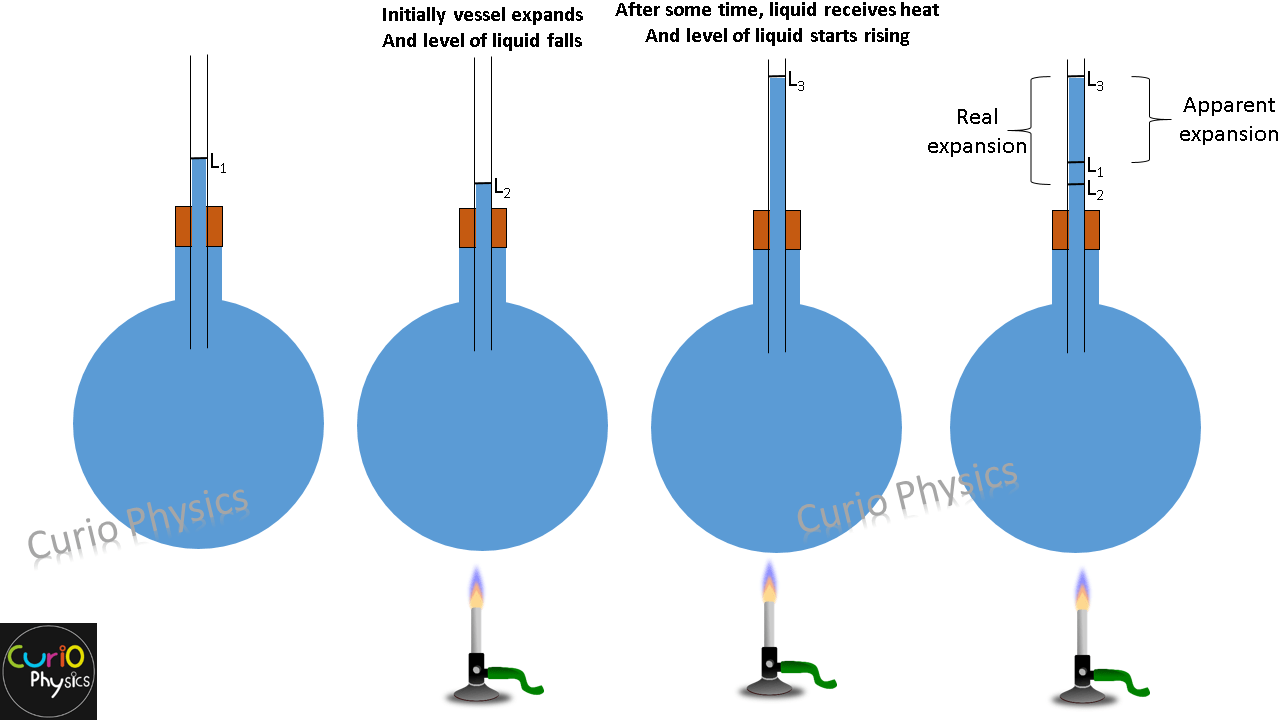# Thermal Expansion of Gases

All three states of matter (solid, liquid and gas) expand when heated, but thermal expansion of gases is much greater than solids or liquids, i.e., on heating, gases expands much greater than solids or liquids.

But change in volume of gases not only depends on change in temperature but also on change in pressure of the gas. This can be understood by following example :-

Suppose we have a bottle which is completely filled with water and its cap is tightly sealed. Now if we increase the pressure on the walls of the bottle in order to crush the bottle then we will not be able to do that. This implies that  pressure (P) and volume(V) of liquids are not related to each others. On the other hand we can easily crush an empty (filled with air) bottle by hands. Meaning that pressure (P) and volume(V) of gases are related.Now to find out volume expansion coefficient γ for gases we have to assume that pressure is constant.

The pressure, volume and temperature of a gas are related to each others by the ideal gas equation :-$\displaystyle PV=nRT$

Considering  pressure (P) constant and differentiating above equation w.r.t. temperature (T) :-$\displaystyle \Rightarrow d(PV)=d(nRT)$$\displaystyle \Rightarrow PdV=nRdT$$\displaystyle \Rightarrow \frac{dV}{dT}=\frac{nR}{P}$

Dividing  both sides by volume V,$\displaystyle \Rightarrow \frac{1}{V}(\frac{dV}{dT})=\frac{nR}{PV}$$\displaystyle \Rightarrow \frac{1}{V}(\frac{dV}{dT})=\frac{nR}{nRT}=\frac{1}{T}$

[because PV=nRT]

By definition, the coefficient of volume expansion$\displaystyle \gamma =\frac{1}{V}(\frac{dV}{dT})$

Hence,$\displaystyle \gamma =\frac{1}{T}$

⇒ Coefficient of volume expansion (γ), for gases depends on absolute temperature of the gas and is independent of the nature of the gas. Which means that equal volume of different gases expand equally when heated by the same amount.

Also note that$\displaystyle \gamma =\frac{1}{T}$, implies that γ for gases is inversely proportional to temperature T. It means gases expand more at lower temperature than at higher temperature.

Note :- For gases coefficient of volume expansion (γ), can be calculated mathematically by taking the reciprocal of the absolute temperature of the gas. But for solids and liquids,  (γ) is found experimentally.

Conclusion :-

1. While dealing with the coefficient of volume expansion of a gas the pressure (P) is assumed constant.
2. All gases having same volume have same coefficient of volume expansion for same rise in temperature.
3. The coefficient of volume expansion of a gas at constant pressure is given by$\displaystyle \gamma =\frac{1}{T}$#### Manoj Kumar Verma

Hi, I'm Manoj Kumar Verma, a physics faculty having 7 years of teaching experience. I have done B.Tech (E.E.). I am also a YouTuber and Blogger. This blog is dedicated to help students learn the physics concepts easily.

View all posts

•Mahender ostwal says:
•Manoj Kumar Verma says: